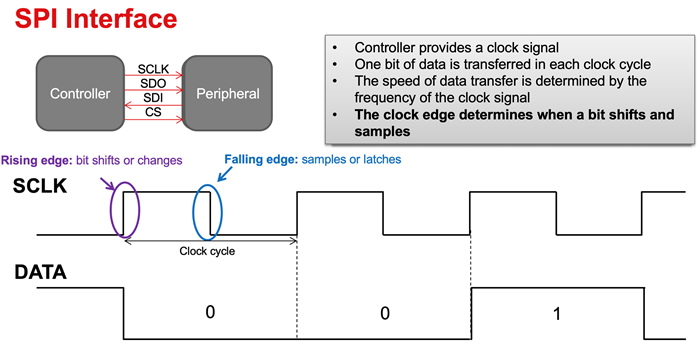If you have a related question, please click the "Ask a related question" button in the top right corner. The newly created question will be automatically linked to this question.

# [FAQ] How to calculate the maximum SPI speed supported by a digital isolator?

Other Parts Discussed in Thread: ISO7741

How to calculate the maximum SPI speed supported by a digital isolator?

• Serial Peripheral Interface (SPI) is a single-master, 4-wire, synchronous, serial communication interface and the 4 signals involved are - SCLK, SDO (PICO), SDI (POCI) and CS\. The controller provides the clock, SCLK, to all the peripherals and one bit is transferred in each clock cycle. Hence, speed of SPI interface is determined by the frequency of SCLK.

During the rising edge of SCLK, data changes to value that needs to be transmitted and during the falling edge it remains stable so that it can be sampled. This is shown pictorially in the below diagram.The below diagram shows a typical SPI connection between an SoC and an MCU through a quad-channel digital isolator. The below diagram also shows how propagation delay shifts SCLK as it passes through digital isolator and how it affects data that is being received.The peripheral prepares data at the rising edge of SCLK and controller samples the data at the falling edge of SCLK. Since data needs to be read in half SCLK cycle and SCLK / SDOI together go through a round-trip propagation delay of digital isolator, the minimum SPI clock period or the maximum SPI speed can be expressed as shown below.

2 * tpd(max) < tSCLK(min) / 2

In other words,

fSCLK(max) < 1 / [ 4 * tpd(max) ]

As an example, let us consider ISO7741 that has a tpd(max) of 16ns for VCC1 = VCC2 = 5V. The maximum SPI speed that can be achieved on ISO7741 is, fSCLK(max) = 15.6 MHz.